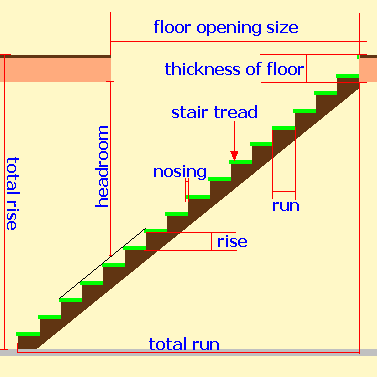Spike's Calculators

# Stair Design Calculator

This calculator calculates riser height from total height and number of risers and provides results for slope, rise and run ratio's, total run of the stairs, stringer length and the minimum required floor opening.### Stair Calculator

Total Rise ft in n
d
Number of Risers #
Run in n
d
Dimension of Nosing in n
d
Floor Thickness in n
d

#### Results:

 Total Rise in Inches in Height of Riser in Stair Slope ° Rise plus Run Equals in Two Rise plus One Run Equals in Rise Times Run Equals in Total Run ft in Stringer Length ft in Floor Opening ft in

#### The Calculation

1. enter the total rise from floor to floor
2. number of risers you wish to calculate for
3. unit run ( length of one run)
4. nosing, the amount the stair tread projects passed the vertical riser below
5. thickness of floor structure (floor finish material plus joist plus plywood plus ceiling finish)
6. required headroom, as per building code / or the desired headroom

#### The Results

1. the total rise in inches
2. the height of one riser
3. the slope of the stairs
4. sum of ratio
5. sum of ratio
6. sum of ratio
7. the total run of your stairs
8. stringer length
9. floor opening size